# c程序实验报告

???1、用for语句实现循环
???2、用while循环语句实现循环
???3、用do-while语句实现循环
???4、用while语句和for语句配合实现循环
???5、用for语句嵌套实现循环

## 1.用for语句实现循环

● 掌握c语言逻辑运算和关系运算的规则
● 掌握for语句实现循环的方法
● 循环嵌套的使用

## 2.用while循环语句实现循环

● 掌握while语句实现循环的方法
● 进一步掌握while语句实现循环的方法

## 3.用do-while语句实现循环

● 掌握do/while语句实现循环

## 4.用while语句和for语句配合实现循环

●掌握while语句和for语句配合实现循环

## 5.用for语句嵌套实现循环

● 掌握for语句嵌套实现循环的方法
● 掌握for语句嵌套（3层）实现循环的方法
● 掌握continue语句的用法

## 1.实验练习：5.3.1

### 流程图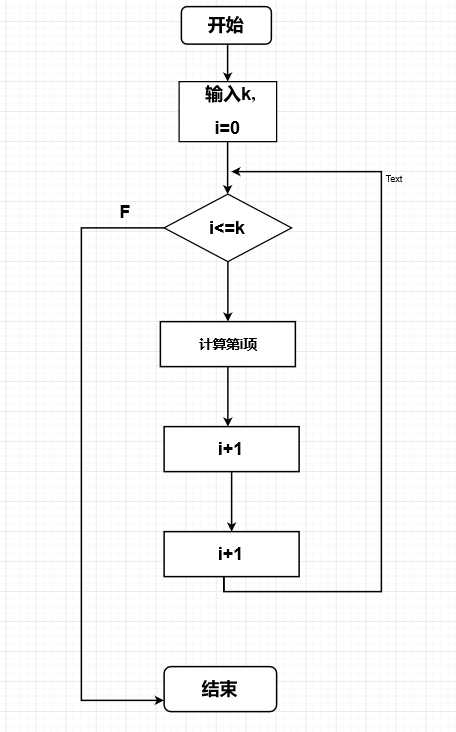### 实验代码

``````#include<stdio.h>
#include<conio.h>
main()
{
int i,j=0,k,s=0;
printf("Enter a number:");
scanf("%d",&k);
for(i=1;i<=k;i++)
{
s=s+j;
printf(" %d",s);
j++;
}
}``````

### 运行结果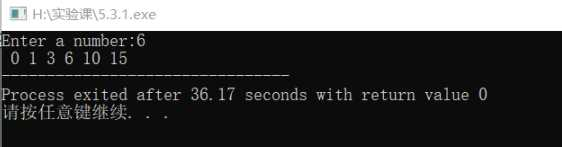## 2.实验练习：5.3.1（2）

### 流程图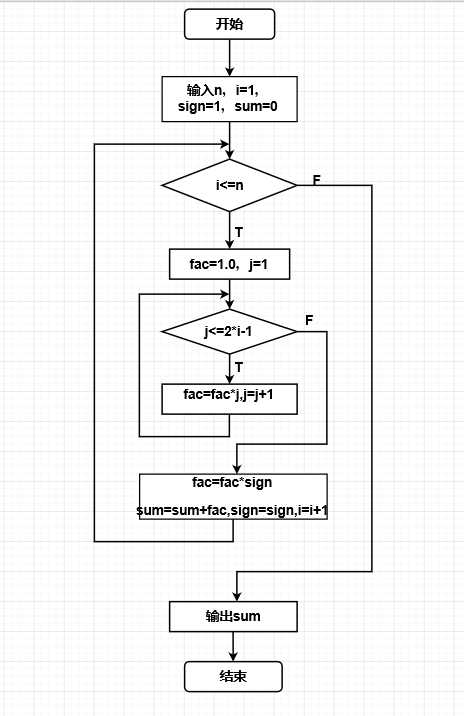### 实验代码：

``````#include<stdio.h>
main()
{
int n,i,j,sign=1;
float fac,sum;
printf("please input value of n:");
scanf("%d",&n);
sum=0.0;
for(i=1;i<=n;i++)
{
fac=1.0;
for(j=1;j<=2*i-1;j++)
{
fac=fac*j;
}
fac=fac*sign;
sum=sum+fac;
sign=-sign;

}
printf("sum=%.0f\n",sum);
}``````

### 运行结果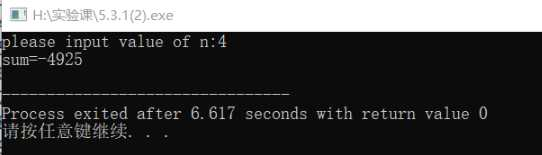## 3.实验练习：5.3.2

### 流程图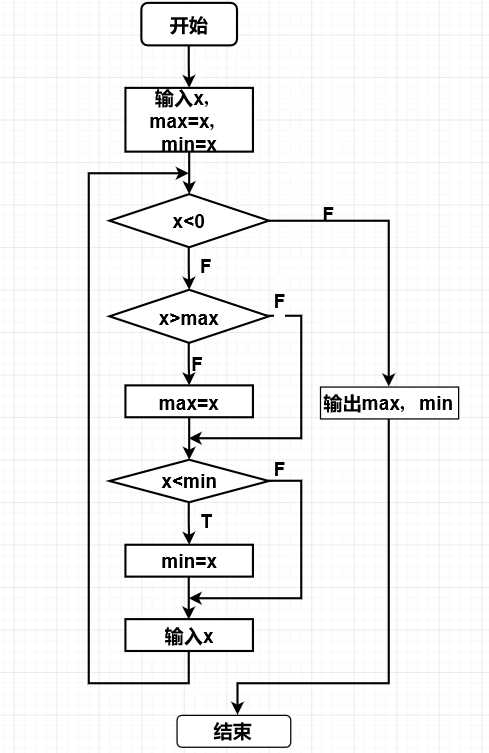### 实验代码

``````#include<stdio.h>
#include<conio.h>
main()
{
float x,max,min;
printf("please input scores:");
scanf("%f",&x);
max=min=x;
while(x>0)
{
if(x>max) max=x;
if(x<min) min=x;
scanf("%f",&x);
}
printf("\nmax=%f\nmin=%f\n",max,min);
}``````

### 运行结果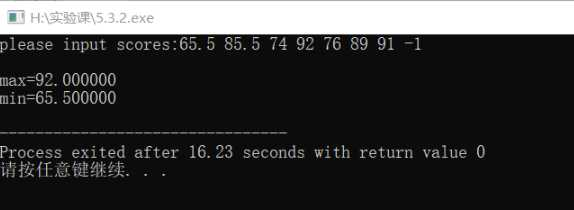## 4.实验练习：5.3.2(2)

### 流程图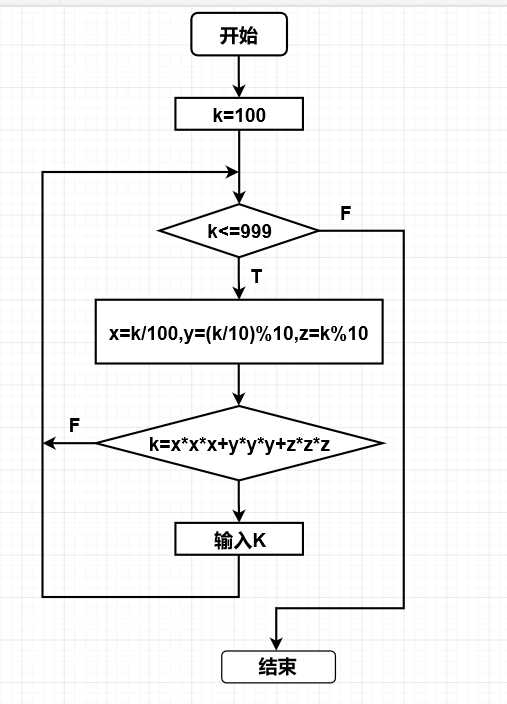### 实验代码

``````#include<stdio.h>
main()
{
int x,y,z;
int k=100;
while(k<=999)
{
x=k/100;
y=(k/10)%10;
z=k%10;
if (k==x*x*x+y*y*y+z*z*z)
printf("%d\n",k);
k++;
}
}``````

### 运行结果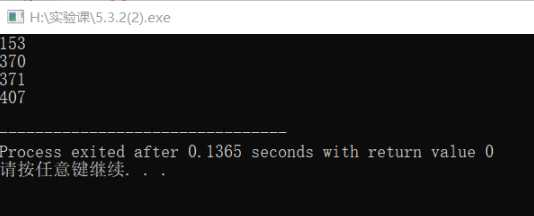## 6.实验练习：5.3.3

### 流程图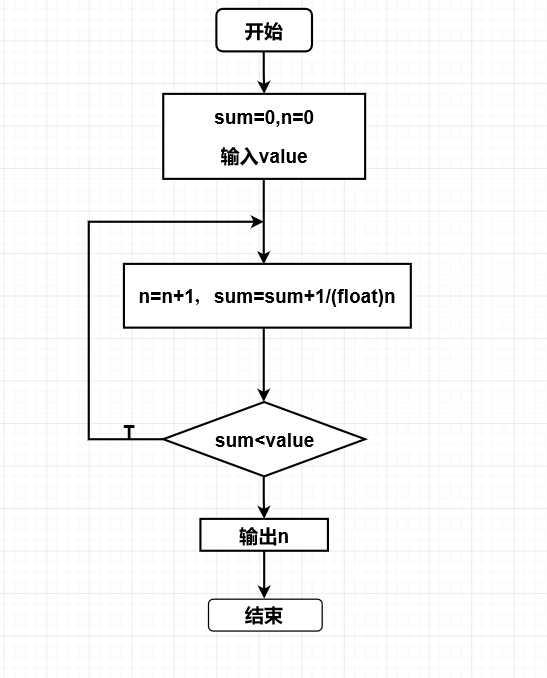### 实验代码

``````#include<stdio.h>
main()
{
float sum,value;
int n;
printf("Input value:");
scanf("%f",&value);
sum=0.0;
n=0;
do
{
++n;
sum+=1.0/(float)n;
}
while(sum<value);
printf("n=%d",n);
}``````

### 运行结果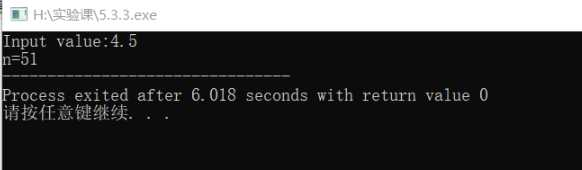## 7.实验练习：5.3.4

### 流程图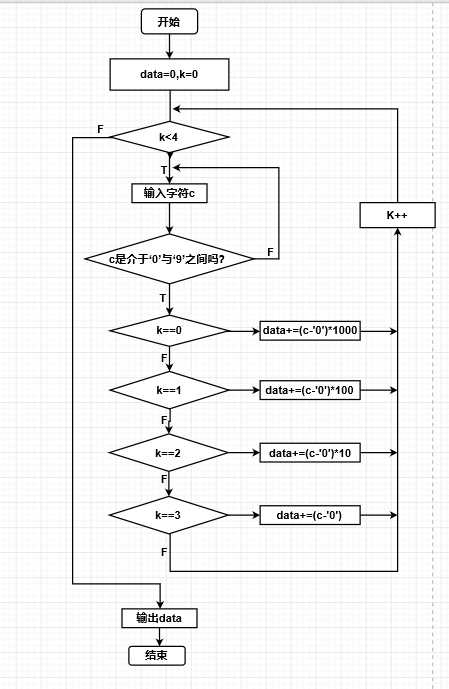### 实验代码

``````#include<stdio.h>
main()
{
char c;
int k,data;
data=0;
for(k=0;k<4;k++)
{
while(1)
{
c=getchar();
if(c>='0'&&c<='9')
break;
}
if(k==0)
data+=(c-'0')*1000;
else if(k==1)
data+=(c-'0')*100;
else if(k==2)
data+=(c-'0')*10;
else if(k==3)
data+=(c-'0');
}
printf("Data=%d",data);
}``````

### 运行结果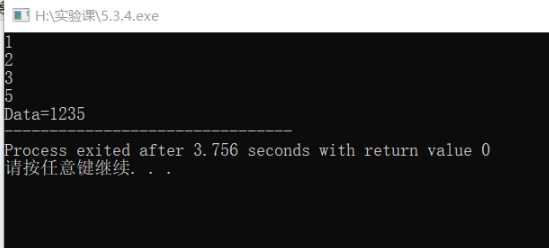## 7.实验练习：5.3.5

### 流程图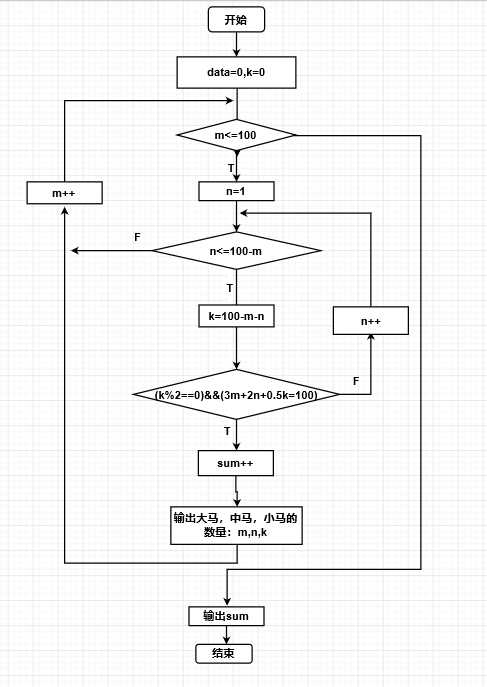### 实验代码

``````#include<stdio.h>
main()
{
int m,n,k;
int sum=0;
printf("各种驮法如下:\n");
for(m=1;m<=100;m++)
for(n=1;n<=100-m;n++)
{
k=100-m-n;
if((k%2==0)&&(3*m+2*n+k/2==100))
{
printf("大马%3d匹;中马%3d匹;小马%3d匹。\n",m,n,k);
sum++;
}
}
printf("共有%d种驮法。\n",sum);
}``````

### 运行结果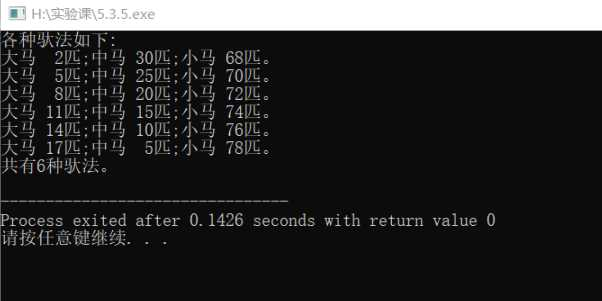## 7.实验练习：5.3.5(2)

### 流程图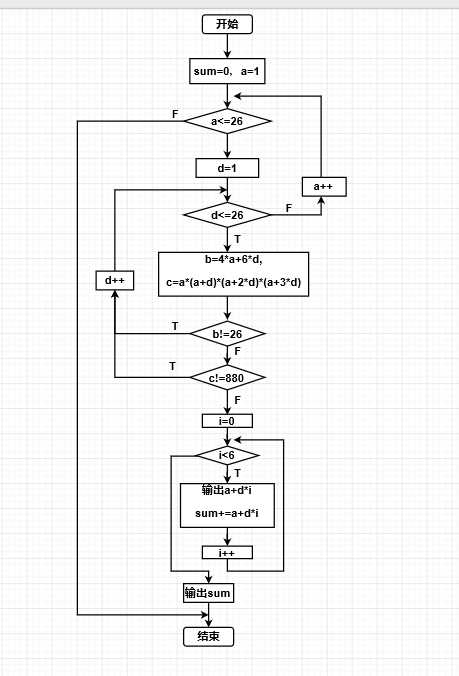### 实验代码

``````#include<stdio.h>
main()
{
int a,b,c,d,i,sum=0;
for(a=1;a<=26;a++)
for (d=1;d<=26;d++)
{
b=4*a+6*d;
if(b!=26)
continue;
else
{
c=a*(a+d)*(a+2*d)*(a+3*d);
if(c!=880)
continue;
else
for(i=0;i<6;i++)
{
printf("%d,",a+i*d);
sum=sum+(a+i*d);
}
}
}
printf("\n数列的前6项的和：%d\n",sum);
}``````

### 运行结果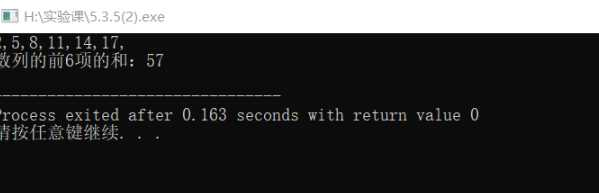## 7.实验练习：5.3.5(3)

### 流程图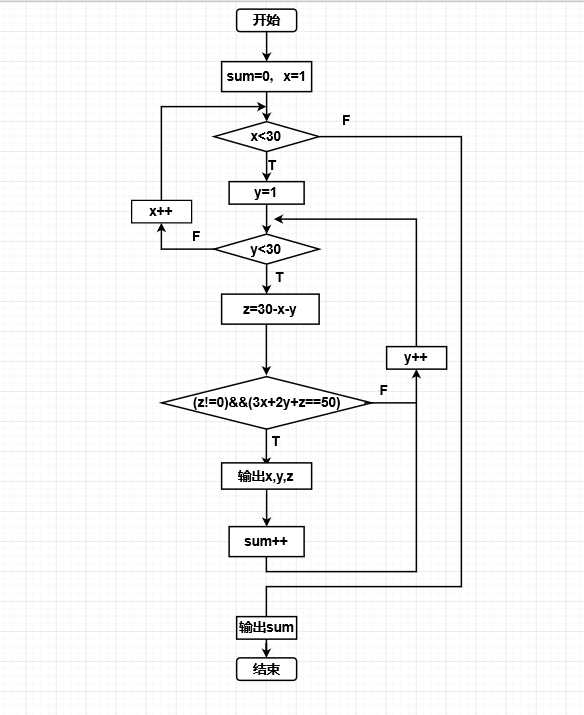### 实验代码

``````#include<stdio.h>
main()
{
int x,y,z,sum;
sum=0;
for(x=1;x<30;x++)
{
for(y=1;y<30;y++)
{
z=30-x-y;
if ((z!=0)&&(3*x+2*y+z==50))
{
printf("大学生%3d\t中学生%3d\t小学生%3d\n",x,y,z);
sum=sum+1;
}
else
continue;
}
}
printf("sum=%d\n",sum);
}``````

### 运行结果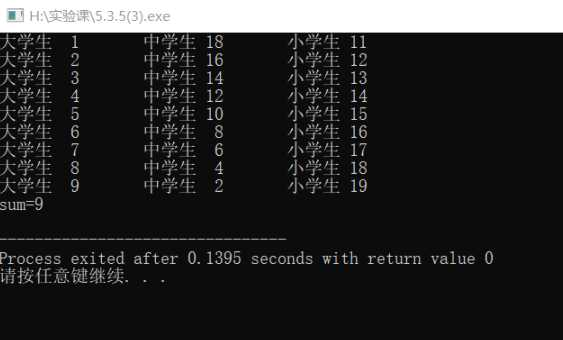## 三、实验小结

(0)
(0)

0条

© 2014 bubuko.com 版权所有 鲁ICP备09046678号-4